Elliott Sound Products Audio Designs With Opamps

# Designing With Opamps - Part 1

© 2000 - Rod Elliott (ESP)
Updated Oct 2019Articles IndexMain Index

Contents
Introduction

There are few audio frequency designs today that do not use operational amplifiers (op-amps, or just 'opamps').  Over the years, the poor opamp has been much maligned, with mainly spurious claims about 'audibility', distortion, and other so-called defects.  There are even people who will compare the bass performance of opamps, which is (IMO) lunacy - all function perfectly to DC, and none will be found to be lacking in low frequency performance (i.e. no loss (or gain) of bass from any opamp with the same feedback resistor and coupling capacitor configuration).

Note that although the term 'audio' is used throughout this series, it doesn't necessarily mean audio in the traditional sense.  Countless industrial processes operate within the same frequency range, so when you see the term 'audio', it generally means 'audio frequency' - and covers the range from DC up to perhaps 30-40kHz or so.

Some of the more basic opamps do have limitations which make them less than desirable in some cases, but most of the new breed are unsurpassed for linearity, with total harmonic distortion figures as low as 0.00003%.  This can be important for industrial processes as well as hi-fi, because very high linearity also means the potential for very high accuracy.  Even the most basic types still have their uses in simple low-speed control circuits and other non-demanding applications.

The operational amplifier was first used in the 1930's as the basis of analogue computers, and much development took place to design accurate gun aiming systems during the Second World War.  Since integrated circuits were unknown at the time (this was before the invention of the transistor), the earliest versions were made using valves.  The basic concept is to have an amplifier with differential inputs, thanks to Alan Blumlein who patented the circuit we now call a 'long tailed pair' in 1936.  The ultimate goal was a circuit whose operation is controlled only by the external feedback components.  By rearrangement of the feedback circuit, different 'operations' could be performed.  Typically, these early opamps could add and subtract, and these are essential functions to this day (even in audio).  One of the earliest commercial opamps was the K2-W made by George A Philbrick Researches (GAP/R), which used a pair of 12AX7 valves, a differential input stage and cathode follower output.  For more information on the early history of opamps, see References.

With the advent of the IC and mass production techniques, the opamp became very popular and remains so - with considerable justification.

This article will concentrate mainly on audio (including hi-fi) applications, but there are some configurations that are just so wonderful that I cannot resist the temptation to include them.  For the most part, any of the configurations shown can use the simplest (and cheapest) opamp you can get (especially for testing), unless extremely wide bandwidth or low noise is a prime consideration.  For any of the test circuits this is not an issue.

I also suggest that you build up the Opamp Design and Test Board (Project 41), which is ideal for the experimenter.  Most of the circuits shown can be built using this test board, and will function perfectly, although there will be limitations as to bandwidth and noise because of the LM1458 dual opamps recommended for the project.  This recommendation is for a purpose - if fast opamps are used, many circuits will oscillate because of long tracks (and wires) from inputs and outputs.

1 - Essential Formulae

To understand this article, you need to know Ohm's law and its derivatives.  Ohm's law is fundamental to electronics, and with little more it is possible to derive most of the other resistance based formulae.  Ohm's law states that a potential of 1 Volt through a resistance of 1 Ohm will cause a current of 1 Ampere to flow.  This is expressed as:

R = V / I ...   where R is resistance, V is voltage and I is current, or ...
I = V / R ...   or ...
V = I × R

Later on, we will also use the formulae for inductive reactance and capacitive reactance, as well as calculating frequency response and some filter design.  These will be presented as needed.  Many people are 'scared' off electronics because they think that high-level maths knowledge is necessary, but for basic circuitry this is not the case at all.  In all cases, I try to keep formulae to the minimum required for a good understanding.  The ESP site doesn't show detailed and complex maths functions unless they are absolutely essential to understand what's going on.

You will see references to 'an instantaneous level of 'x' volts AC'.  At any point in time, an AC voltage has an instantaneous voltage - this is the voltage that is present at that moment, and for analysis can be treated as DC.  This is valid only when we consider this 'DC' level as a transient thing, since many of the circuits do not operate down to DC at all (many others do, but this is beside the point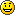).

2 - Basic Rules of Opamps

Many years ago I used to teach electronics, and I devised what I called the 'Basic Rules of Opamps' for the purposes of explanation.  There are two Rules, and although real life is never like theory (I could fill the page with suitable examples, but shall refrain), they describe the operation of all opamp circuits very accurately:

#1     An opamp will attempt to make both inputs exactly the same voltage (via the negative feedback path)
#2     If it cannot do so, the output will assume the polarity of the most positive input

Needless to say, this requires some explanation.  So let's look at Rule #1.

#1     An opamp will attempt to make both inputs exactly the same voltage

When an opamp is operated in its linear mode (which is most of the time for audio and most other amplification circuits), the negative feedback circuit will cause a voltage to appear on the inverting input (-in) that is (almost) exactly equal to that present on the non-inverting input (+in).  Any change of voltage on either terminal is reflected by a change in the output that causes more or less current to flow in the feedback circuit to restore equilibrium.  For negative feedback, there must be a resistance between the opamp's output and its inverting input.  There will normally be a second resistor from the inverting input to set the circuit's gain.  This resistor may go to ground, an 'artificial ground' or used as the input.

If this is unclear to you, see the further explanations below - but remember the 1st Rule!  While it sounds simplistic, it actually describes the linear operation so well that you will rarely need to concern yourself (at least during circuit analysis) with the minor deviations that inevitably occur due to limited gain, input offset voltages, etc.  These are important, but they don't help with understanding what the device is trying to achieve.

#2     If Rule #1 cannot be satisfied, the output will assume the polarity of the most positive input

There are many circuits that use opamps in non-linear mode, and this can also happen if the output cannot swing its voltage fast enough (known as slew-rate limiting).  In these cases, should the +in terminal be the most positive, then the output will swing positive (at its maximum possible speed, aka slew rate).  If the -in terminal is more positive, the output will swing negative.

This condition is usually the result of no (negative) feedback, and may or may not include positive feedback, where the opamp's output is connected (via a resistor) to the non-inverting input pin.  Positive feedback is not a requirement for Rule #2 though, it's entirely optional, depending on what the designer wants to achieve.

There is almost no opamp circuit that you cannot understand once these Rules are firmly established in your thinking.  Even circuits that use external transistors in strange ways will obey the Rules.  An opamp that does not perform as above is being used outside of its normal operating parameters, and the results will be unpredictable and almost always unsatisfactory.

It is often explained that an opamp reacts only to the difference between the two inputs, and not to their common voltage (common mode voltage is any voltage that appears on both inputs when the circuit is in equilibrium).  While essentially true, this doesn't have the absolute clarity of 'The Rules', nor does it help general understanding.  The ability to ignore the common voltage is called the Common Mode Rejection Ratio (CMRR), and will be covered later in this article.

3 - Some Essential Opamp Information

Before we cover the circuits themselves, we need to look at some of the parameters you will come across, how to apply power and bypass the supply rails and so on.  There are many parameters that you will see in data sheets, and these are covered in more detail a little later.  There is no point doing it now, as the importance will be lost until you know more about the opamp itself.

3.1 - Configurations

Opamps come in a variety of configurations, but the most common are:

Single - one opamp in an 8 pin DIL (Dual In Line) or SOP (small outline (SMD) package)
Dual - Two separate opamps, sharing only the power supply pins - commonly in an 8 pin DIL or SOP (may also include SIP - single inline package and 14 pin packages)
Quad - Four separate opamps, again sharing the power supply - most commonly in a 14 pin DIL or SOP

Amazingly, nearly all opamps use the same pinouts, and these were established many years ago by the venerable µA741 for single opamps, and the likes of the LM1458 dual opamps set the stage for the others that followed.  Many of the quads use the same pinouts as well, and this has enabled people to swap opamps for 'better' ones for a very long time.  There are some different terms used for SMD (surface mount device) packaging, including SOP, SOIC, MSOP and various others.  The available styles and dimensions are available in the datasheet for the opamp you want to use.

However - Don't count on complete standardisation!  There are some variations, and although uncommon, they do exist.  I shall not be concerned with any of the different devices - only the common pinout versions will be shown.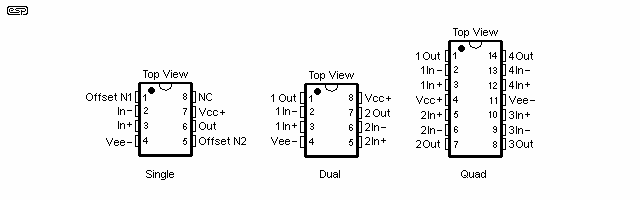Figure 1 - Common Opamp Pinouts

Figure 1 shows the standard connections for single, dual and quad opamps, but be aware that the remaining pins on the common single devices can occasionally have uses other than those shown.  The additional connections available are most commonly:

• Offset Null - used to adjust the amplifier to ensure that the input transistors are perfectly balanced, so that with no input signal, there is zero volts output.  This is important for DC amplifier circuits.
• Compensation - Some single opamps do not have inbuilt frequency compensation capacitors, and these are connected externally instead.  This allows the designer more freedom, and the opamp's high frequency performance can be optimised for the design objective.

You may at times see these connections used in unconventional ways.  This may be to obtain greater bandwidth than might normally be available, or perhaps just so the designer can show how clever s/he is.  Either way, I shall not be delving into these aspects of the design process.  Some opamps are compensated for some specified minimum gain.  For example, the NE5534 (single opamp) is stable with a gain of three (10dB) or more without adding a compensation capacitor.  If the required gain is less than three, external compensation is required to prevent oscillation.

3.2 - Applying Power

No (active) circuit works without power, so this has to be the first step.  Most opamps will operate with a maximum of 36V between the supply terminals.  As this is the absolute maximum, operation at a lower voltage is the general rule, and the most common is to use ±12V to ±15V to power the circuit.  Some opamps are rated for higher voltages, and others for less, so consult the spec sheet from the manufacturer.  There are also many new devices that are designed for 5V operation (or ±2.5V) and these will die if run from any voltage greater than the absolute maximum voltage specified.

A dual supply is not required, but it does simplify the design and is recommended for most applications.  A dual supply has the advantage that all inputs and outputs are earth (ground) referenced.  This can eliminate a great many capacitors from a complex design, and is the most common way to power most opamp circuits.  Note that from a commercial perspective, elimination (or reduction) of capacitors is done for economic reasons rather than any great desire to 'simplify' the signal path or eliminate 'evil' capacitors.

Since the pinouts are nearly always the same, Figure 1 will be applicable in most cases, but as I said earlier "Don't count on it!".  When in doubt, get the specification sheet from the manufacturer.  When not in doubt, get the specification sheet anyway.

Power Supply Rejection Ratio
The PSRR (Power Supply Rejection Ratio) of an opamp is a measure of the amount of power supply noise that finds its way into the output signal.  Most specification sheets give the test conditions for this measurement, and this should be consulted if an unusual design is contemplated.  Mostly it can be ignored.

Bypassing
Although most opamps have a very good PSRR, this cannot compensate the IC for power supply lead (or track) inductance, and this can cause serious misbehaviour of the opamp in use.  It is always recommended that the supply be bypassed with capacitors - with special attention needed with high speed opamps.  Bypassing should always use capacitors with good high frequency performance, and multilayer (aka monolithic) ceramics are the best in this regard.  It is common for designs to use electrolytic capacitors, themselves bypassed by low value (100nF) capacitors.  This ensures that all trace inductance is properly 'neutralised', and helps to prevent oscillation.  When this occurs with a high speed (HS) opamp, it will commonly be in the MHz region, and may be extremely hard to see on basic oscilloscopes.

A sure sign of oscillation is inexplicable distortion, that mysteriously disappears (or appears) when you touch the opamp or a component in its immediate vicinity.Figure 2 - Bypassing The Opamp Supplies

Even with HS opamps, electrolytic capacitors are (usually) not needed for each device (generally needed only on each board), but the use of ceramic bypass caps between the supply pins of each device is highly recommended.  Figure 2 shows a common method of bypassing power supplies for opamp circuits ('A'), but there are others.  In some cases, the supplies may not be bypassed to earth (ground), but just to each other.  This has the advantage of not coupling supply noise into the earth (ground) system ('B').  The approach I usually take with PCB designs is shown in 'C', with a pair of electros at the point where the DC is connected to the PCB, and a bypass cap between the supplies of each opamp (or opamp package).

Claims have been made that supply bypassing ruins the sound (rubbish), or that ceramic caps should never be used in audio, even for bypassing (more rubbish), and even that high value capacitors (> 1µF) slow down the sound (unmitigated drivel).  These claims are often made by frauds and charlatans, then perpetuated by unwitting hobbyists and others who don't know enough to be able to perform detailed analysis.  Claims like this should be completely ignored - they have no basis in fact whatsoever, and indeed, quite the reverse may be true in each case.

Note that bypassing alone is not sufficient to ensure stability under all conditions.  Poor PCB layout can create problems too, and it's often necessary to take extra precautions with the layout to avoid issues that can be extremely difficult to track down.  This is doubly true for inexperienced designers who are unaware of the general 'risk factors'.  You will know that you have a layout or bypassing problem if a slow opamp works fine, but a faster one oscillates or causes severe ringing on transient signals (including squarewaves).  A common error is to omit an output resistor (typically 100 ohms) to isolate the opamp's output from capacitive loads such as coaxial cables (including standard RCA interconnects).

3.3 - The Ideal Opamp

An ideal opamp has an infinitely high input impedance, and therefore needs no bias current.  It is also capable of infinite gain without feedback, so there are no errors between the two inputs (i.e. Rules 1 & 2 will hold for all cases).  The ideal opamp also has infinite bandwidth, no internal delay, and zero ohms output impedance.  It is capable of supplying as much current as the load can draw, without the voltage being reduced at all.  The ideal opamp does not exist.

Although it does not exist, the ideal opamp is the common model for nearly all opamp circuits, and few errors are encountered in practice as a result of designing for the ideal, and actually using a real (non-ideal) device.  The tolerance of even the best resistors will ultimately limit the accuracy of any opamp circuit at low frequencies (where gain is highest).  This does not mean that any opamp can be used in any circuit - the designer is expected to be able to determine the optimum device for the task.

Although most circuits show the opamp's output referred to the system common (typically earth/ ground), the opamp itself has no intrinsic reference - the design sets the reference, not the opamp.  During the design phase, one of the tasks of the designer is to set up the reference, which is simply a connection that's common to both the input and the output.  It only has to be within the bounds set by the power supplies and the device itself.  When using (say) ±15V, the common is usually zero volts (ground).  Depending on the design, it could be some other voltage - the opamp doesn't care as long as it's used within datasheet specifications.

The primary practical limitations of real-world opamps are as follows:

• Input Impedance - Typically from one to several hundred Megohms.  FET inputs are used for very high impedance inputs (1TΩ is not uncommon)
• Gain - 100-150dB from DC up to a few Hertz is normal, then rolling off at 6dB/ octave (20dB/decade), some have more gain at higher frequencies ¹
• Common Mode Input Voltage - typically limited to the supply voltages, but may be up to 0.6V greater (or less for the negative swing) with some ICs
• Bandwidth - opamps with a usable high frequency limit of 1MHz at unity gain are now common, and some extend a lot further (100MHz or more)
• Output Current - most common opamps are limited to about 20mA of output current, although some can provide more (up to 50mA for example)
• Slew rate - how fast the output can change, in V/µs.  Ranges from 0.5V/µs to 20V/µs (some are faster, but can also be harder to use)
¹     This applies especially when high frequency compensation is external, and/or the opamp is specified for a minimum gain (meaning the device cannot be used as a unity gain voltage follower)

There are others, such as input offset voltage and current, but we shall not concern ourselves with these parameters just yet.  Power opamps (IC power amplifiers) may be capable of up to 10A, but these are outside the scope of this section of the article.  They don't qualify as being 'true' opamps, but they behave in a very similar manner and have similar input and output requirements and/or limitations (depending on output power).

The use of ideal opamps is assumed for much of the following, but all are designed to function properly with real world devices.  In practice the difference between an ideal opamp and the real thing are so small as to be ignored, but with one major exception - bandwidth.  This is the one area where most opamps show their limitations, but once properly understood, it is quite easy to maintain a more than adequate frequency response from even basic opamps.

The common mode input voltage can be important in some applications.  Ideally, an opamp only reacts to the voltage difference between its inputs.  Provided this does not change, in theory, the actual voltage between the two inputs and the common (zero volt line) may be anywhere within the specified range with no change in the output voltage.  In other words, the inputs can assume any voltage between the negative and positive supplies, and there will be (almost) no change at the output.

With a real (as opposed to ideal) opamp, there will be some change, and this is specified as the common mode rejection ratio.  An opamp with a CMRR of 100dB (not uncommon) will ensure that the change in output voltage is 100dB less than the change of input voltage (as applied to both inputs simultaneously).  Any difference between the inputs is amplified normally.  CMRR is affected by the open loop gain of the opamp, so is usually worse at high frequencies.  High common mode voltages can adversely affect distortion performance, but rarely to the point of it becoming audible.

While Rule #1 states that the opamp will try to make both inputs the same voltage, this can only apply if the opamp's gain is infinite.  If an opamp has a gain of 100dB (100,000 times), then the input voltage difference will be 1/100,000th of the output voltage.  If there's 1V at the output (for a unity gain circuit), the difference between the inputs will be 10µV.  While this is very real, it can be ignored in 99% of common applications.  Rule #1 remains valid unless you are trying to make the opamp do something 'interesting'.

4 - The Basic Opamp Circuits

The following collection shows the most common configurations for amplifiers.  These are intended as linear amplifiers, in that they are essentially distortion free (within the capabilities of the opamp itself, of course).

As we progress, most of these original circuits will be seen over and over again, since they are the very foundations of building an audio circuit using opamps.

In all cases, a dual power supply is assumed, and this is not shown on the circuits.  This partly for clarity, since the additional circuitry makes the diagrams harder to understand, and partly because it is a convention not to show all the supply connections anyway.  We all know they have to be there, so there is little point in showing the obvious over and over again.  Likewise, bypass capacitors and other support components are not shown - only the basic opamp and its associated components.

You will also see reference to the 'instantaneous value of the AC waveform'.  This is like a snapshot, and we simply freeze time while we analyse the operation of the circuit.  At any point in an AC waveform, it can have only one value of voltage and current, regardless of the complexity of the signal source.  A sinewave is no different from any other signal - provided its amplitude and frequency are within the capabilities of the opamp.

4.1 - The Non-Inverting Amplifier

The most common of all configurations is the non-inverting amplifier.  I will therefore use this as a starting point, because it is also the simplest to understand.  Figure 3 shows a completely conventional non-inverting opamp voltage amplifier.Figure 3 - Non-Inverting Opamp Amplifier

Rin is the input resistor, and is needed because an opamp needs a reference voltage at the input.  In this case the reference voltage is the zero volt (earth) bus.  Input impedance is equal to the value of Rin in parallel with the opamp input impedance.  Generally the latter can be ignored because it is so high.

The gain (Av - Amplification, Voltage) is set by the ratio of R1 and R2, and is equal to:

Av = ( R1 + R2 ) / R2     (or R1 / R2 + 1 )
The gain of this stage cannot be less than unity, regardless of the resistor values used.  As shown in the diagram, the gain is 11 times, so a 100mV input will become a 1.1V output.  To re-examine Rule #1, it is obvious that if 100mV (instantaneous AC or DC) appears at +in, the amplifier must have 1.1 volts at the output, since the voltage divider R1/R2 will ensure that 100mV also appears at -in.  This is obtained from the simple voltage divider formula, which is strangely familiar ...
Vd = ( R1 + R2 ) / R2     (or R1 / R2 + 1 )

This will hold for any gain and any output within the capabilities of the power supply and the opamp's design.  A signal at 10MHz will not follow the rule, since the opamp will almost certainly be incapable of amplifying such a high frequency.  An input voltage of 10V with a gain of 11 will also break the rule, since the opamp has only ±15V supplies, and the output cannot exceed the supply voltage.  Likewise, an 8 ohm load will break the rule, since the opamp cannot supply the current needed to drive such a load.

To see how the opamp behaves in these abnormal conditions, I suggest that the circuit be built, and run the tests if you have access to an oscilloscope.  Examine the inputs as well as the output, since the inputs are by far the most interesting when the opamp is appearing to break the Rules.

4.2 - Inverting Amplifier

Once, all amplifiers were inverting.  A single valve or transistor stage (other than a cathode or emitter follower buffer stage) always inverts the signal, and this is how it must be (see Amplifier Basics - How Amps Work for more info).

With the advent of the opamp, all this changed, and the inverting amp is a very different beast from the simple discrete designs.  The gain ratio is again set by a pair of resistors, but the +in terminal is earthed, either directly, or via a resistor.  This configuration is also called a virtual earth (or virtual ground) stage, and is common in mixing consoles and many other signal processing circuits.

When used in this mode there is both an advantage and a disadvantage.  The advantage is that there is no common mode signal at the inputs because the two inputs will be at (close to) zero volts.  All opamps have some additional distortion with high common mode voltages, and while it's rarely a real problem, it can reduce performance if you need ultra-low distortion.  The disadvantage is that the circuit has a higher 'noise gain' than an equivalent non-inverting stage.  For a unity gain buffer, the noise will be double that of a non-inverting stage.  Noise gain is equal to R2 / R1 +1.  Inverting stages should never be used for ultra low noise circuits.Figure 4 - Inverting Amplifier

Since +in is earthed and Rule #1 says that both inputs must be the same, -in will 'appear' to be at earth potential as well (i.e. zero volts).  Assume an input of 100mV DC.  The output will be at -1V DC, a gain of -10 (the minus indicates only that it is inverting, not that the circuit has 'negative gain' which is actually a loss).

Input impedance is equal to the value of R1, and voltage gain is R2/R1, or 10 as shown.  Note that this configuration is capable of negative gain (loss).  If R1 is larger than R2 (say 20k), then the gain is equal to R2/R1 as before, so is now -0.5.

To verify that the gain equation works, look at an input at 100mV (again instantaneous AC or DC).  The input current will be 100mV / 1k (using Ohm's law), which is 100µA.  The current through the feedback resistor must be exactly equal and opposite to ensure that zero volts is at the -in terminal (so we don't break Rule #1).  As it happens, -1V / 10k gives us -100µA, the currents cancel, and the requirements are satisfied since the output is negative.

As before with the non-inverting amp, the limitations of the opamp and its supply may cause Rule #1 to be broken, but the amp is now no longer operating in its linear mode, and Rule #2 will take over.  Observation of the -in terminal will show a distorted waveform when the opamp can no longer operate in linear mode.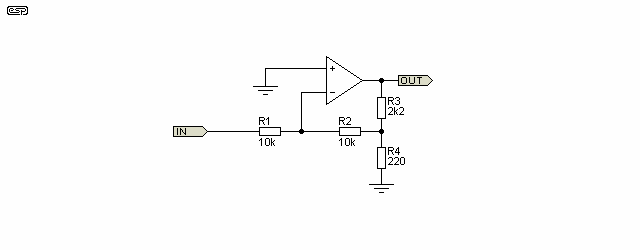Figure 4A - Inverting Amplifier With 'T' Feedback Network

Figure 4A shows an alternative inverting circuit.  Using R3 and R4 means that a higher input impedance can be used, but with a somewhat reduced noise penalty due to (very) high resistances in the feedback circuit.  The circuit shown has a gain of 11.2, and if the Figure 4 circuit were used, the input resistor (R1) would have to be 10k, and feedback resistor (R2) would then be 112k (gain is R2/R1).  The high value feedback resistor creates noise (see Noise In Audio Amplifiers for details).  By using the arrangement shown, resistor values are reduced and so too is their noise contribution.

It is a little harder to calculate the gain.  It's really only a simple formula that can be reconstructed from its constituent parts easily enough once you see (and understand) the relationships.  Assume an input of 1V (peak or DC), and note that R2 is effectively in parallel with R4 (the opamp's input is at zero volts).  Provided R1 is equal to R2, the gain is ...

Av = R3 / ( R4 || R2 ) + 1       Where Av is voltage gain, and '||' means 'in parallel with'
Av = 2.2k / ( 220 || 10k ) + 1
Av = 2.2k / 215.3 + 1 = 11.2       (11.2183 if you want to be exact)

We know that the opamp's inverting input must be at zero volts for linear operation, so the voltage at the opamp's output has to provide exactly 1V to the centre of the 'T' (R2, R3 and R4).  Therefore, the output must be -11.2V to obtain -1V at that point, so the voltage at the inverting input is 1V + -1V = 0V.  While this arrangement is a little more convoluted than just using a 112k feedback resistor, it does provide a worthwhile noise improvement.  This become more important as input impedance and/ or gain are increased further.  There's nothing you can do to increase the input impedance, other than increasing the values of R1 and R2.

It's more irksome to calculate the gain if R1 and R2 are not equal, but it can be done, and I leave it to the reader to figure that out.  In general, there's usually no good reason to make these resistances different, because the majority of the gain will usually be set using R3 and R4.  If a high input impedance inverter is necessary, it's better to use a non-inverting buffer before the inverting stage so all resistance values can be minimised.

Used in the way shown in Figure 4A, the opamp's own noise is amplified by 12.2 and an opamp is always noisier when used in inverting configurations than for a non-inverting circuit with the same gain.

4.3 - Inverting and Non-Inverting Buffers

A very common opamp application is the buffer stage, which (for the non-inverting configuration) can have an extraordinarily high input impedance, and a low output impedance.  As with all opamp circuits, the output impedance may be very low (typically < 10 ohms), but the output current capability will not allow the circuit to drive such an impedance at more than the 20mA or so that is typical of most opamps.  This would limit the output voltage (before clipping) to a maximum of +/-160mV, or about 113mV RMS into 8 ohms.  Distortion will be unacceptably high, and the end result is not worthy of further consideration.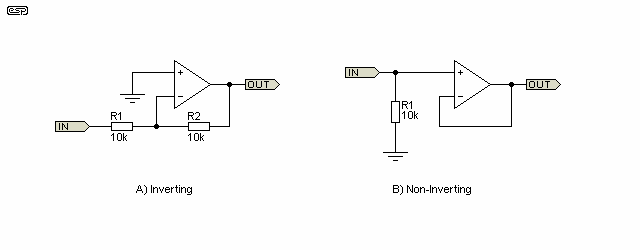Figure 5 - A) Inverting and B) Non-Inverting Buffer

In many cases the non-inverting buffer can be replaced by an emitter or source follower, but performance is nowhere near as good.  Input impedance is lower, output impedance is higher, and the gain is not quite unity.  In addition there is more distortion and lower output drive capability, as well as higher quiescent current.

The inverting buffer is more of a convenience than anything else, and is simply a normal inverting amplifier with unity gain.  Input impedance is the same as R1, and very high values are not possible without excessive circuit noise.  The inverting buffer also suffers from an increased 'noise gain' (amplification of the IC's own internal noise).  This is because the signal has unity gain, but IC input noise has a gain of 2.  In fact, all inverting opamp stages have a noise gain that's equal to the voltage gain plus one.  For example, an inverting stage with a gain of 10 has a noise gain of 11.  Noise is a separate topic, and is discussed in detail in the article 'Noise in Audio Amplifiers'.

5 - Some Interesting Variations On Basic Circuits

It is now time to look at a few of the many variations on the basic circuits discussed above.  It is not possible to cover all the different circuits that have been made using opamps, since there are so many that I could easily end up with the world's longest web page.  I doubt that this would be appreciated by most of you.

I shall only cover the more common, or most interesting, as this will give a better appreciation of how versatile these building blocks really are.  All of the circuits that follow will work - they are not theoretical, but real designs, and can all be made on the opamp test board.

5.1 - High Impedance Amplifiers

The non-inverting buffer has been used in some very interesting ways.  For example, a standard low cost TL071 opamp has an input bias current of about 65pA, and a claimed input resistance of 1012 ohms.  To put this into perspective - assuming we have a way to supply the bias current without affecting input resistance - the input impedance could be as high as 1,000,000,000,000 ohms.  That is 1Tohms (1 Tera-Ohm is 1000 Gig-Ohms ).  We will be completely unable to achieve this in practice, since the insulation resistance of a PCB is nowhere this figure, and the smallest amount of contamination will reduce the impedance dramatically.

In reality, we can easily expect to be able to get an input impedance of 100M ohms or more (I have a project for a 1G ohm test amplifier), but care is needed, since with high value resistors additional noise is produced.  Since noise in a resistor is proportional to the voltage across the resistor and its resistance, it is easy to see how a simple circuit can become a real noise generator.  Figure 6 shows the circuit and PCB layout for a very high impedance amplifier.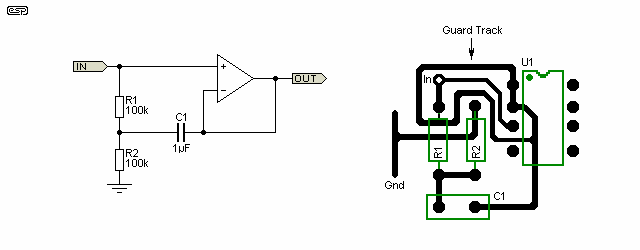Figure 6 - High Impedance Amplifier

The bias resistor is 'bootstrapped' from the output, and this allows a lower resistance while maintaining an extraordinarily high input impedance.  A circuit such as this could be used for a capacitor microphone (for example), which will typically have such a small capacitance that any loading will reduce the low frequency performance to an unacceptable degree.  The guard track can be seen encircling the input and the input end of R1.  What on earth is a guard track?  Read on ....

To prevent the resistance of the PCB from causing a problem, the input section may be 'guarded' with a section of track connected back to the output.  Bootstrapping and guarding work in the same way.  The guard track works by maintaining a voltage from a low impedance source around the input circuit that is the same voltage as the input.  If they are the same voltage, no leakage current will flow.  In reality it is not quite that simple.

Assume that the opamp has 100dB of gain at 1kHz (our test frequency).  This equates to 100,000 - a little shy of infinity!  Since the opamp has a finite gain, the 'unity gain' buffer will actually have a gain of 0.99999 - not 1 as we had assumed.  This error reduces the ability of the opamp to bootstrap the circuit perfectly, so the 100k input resistance will only be effectively increased to 10G ohms.

But wait .... how does it increase the effective resistance at all?  This is very simple.  Assume an instantaneous AC voltage of 1 volt input to the amp.  Normally, this would cause a current of 10µA into the 100k resistor of Figure 6.  Because the bootstrapping action causes the voltage at the junction of R1 and R2 (Fig 6B) to be 0.99999V, there is actually only 1 - 0.99999 = 10µV across the resistor.  The input current is now 10µV / 100k = 100pA (1 pico-amp is 10E-12A).  We can now calculate the equivalent resistance as R = 1V / 100pA = 10G ohms.  This will fall at increasing frequencies as the opamp starts to run out of gain.

Oh yes, the term 'bootstrap' comes from the unlikely picture of a man 'lifting himself off the floor by his own bootstraps'.  As you might have guessed, the term is somewhat antiquated, but there has never been any move to change it (thank goodness).  It is intended to show that the impossible can be done, but it is not really impossible, and is just a very clever example of lateral thinking.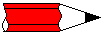The bootstrapped circuit cannot be used at DC, since it requires a capacitor for its operation.  This is not as much of a limitation as may first be thought, since DC is quite inaudible.  However, for some applications, high impedance to DC is a requirement, and then very high resistance values are needed (such as my 1G ohm test preamplifier).

Many common transducers use capacitance as their mechanism.  'Condenser' (i.e. capacitor) microphones are the most common example, but there are many others.  These are normally supplied from a high voltage (50-200V) via an extremely high value resistor.  One would expect noise, but they are usually much quieter than expected.  This is actually easily explained ...

The capacitance may only be small, but the resistor is such a high value (commonly 10M or more) that the transducer itself acts as a filter capacitor.  For example, even a 100pF capacitor makes an excellent low pass filter when fed with an impedance of 100MΩ, having an upper -3dB frequency of 16Hz.  Any noise is effectively filtered out by the capacitance of the transducer.  Remember too that there should be no voltage across the resistor, as that implies that something is drawing current (unacceptable for a capacitive transducer).

However, it must be understood that a bootstrap circuit may have some unintended consequences.  The composite circuit includes the capacitance of the sensor used, and if that changes (by using a different sensor or a longer cable for example) the circuit may either roll off earlier than expected, or show a pronounced response peak at some low frequency that's determined by the feedback components.  The bootstrap circuit is feedback, and by default creates a high-pass filter that may have a very high Q.

This is a topic worthy of an article by itself, and having done many tests with just such a circuit I know only too well that this can create problems.

5.2 - Simulated Inductor

This circuit has to be one of the all-time classics.  Although it can also be done with a single transistor (including JFET or MOSFET), the performance of the opamp version is so much better that the alternative is not really worth considering.

Inductors have always been a problem in electronics, as they are by nature relatively large, and being made from a coil of wire, tend to pick up mains hum as well as other noise in the electromagnetic spectrum.  The idea of simulating an inductor using an opamp has been about for a long time.  The inventor was a Dutch engineer named Bernard Tellegen, to whom we all owe a great debt because it's such a useful circuit.  See Wikipedia for more info.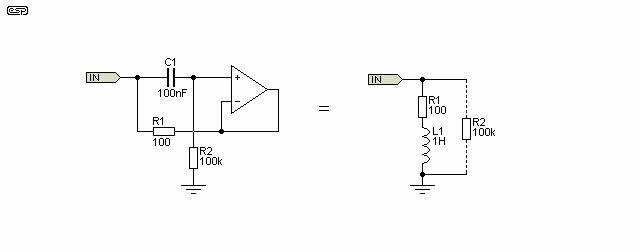Figure 7 - Simulated Inductor

The circuit is much smaller than a real inductor (at least for the larger values), and does not suffer from noise pickup.  It does have a limited Q (quality factor), but it is rare that very high Q circuits are needed in audio, so this is not really a problem.  It is also variable over a moderately wide range, something that is very difficult with the wire wound 'genuine' article.  R2 in parallel with the inductor 'circuit' is rarely shown in 'equivalence' diagrams, but if you want an accurate representation it must be included.  The simulated and real inductors perform identically once the parallel resistance is included.

So, how does it work?

The idea is very simple, but operation is less easy to understand.  Essentially the circuit uses a capacitor, and 'reverses' its operation, thus making an 'inductor'.  The essential character of an inductor is that it resists any change in its current, so if a DC voltage is applied to an inductance, the current will rise slowly, and the voltage will fall until the internal resistance becomes significant.

An inductor also passes low frequencies more readily than high frequencies - the opposite of a capacitor.  An ideal (that word again) inductor has zero resistance, so will pass DC with no limitation, but will have an infinitely high impedance at infinite frequency.  These limits are generally considered to be outside the audio range.

To understand how the circuit works, remember that the output of the opamp is (almost) exactly the same as the non-inverting input.  Imagine a DC voltage of 1V is suddenly applied to the input, via resistor R1.  The opamp will ignore the sudden load because the change is coupled directly to the input via C1.  The opamp will represent a high impedance.  Just as an inductor would do.

With the passage of time, C1 charges via R2, the voltage across R2 falls, the opamp sees less and less of the input signal, and starts to draw current from the input via R1.  This continues as the capacitor approaches full charge, and the opamp has close to zero input, so the output is also close to zero volts.

Eventually resistor R1 becomes the only limiting factor to current flow, and this appears as a series resistance within the inductor in the same way as the resistance of the wire in a real inductor behaves.  This series resistance limits the available Q of both the simulated and real inductor, with the main difference being the magnitude - real inductors generally have less resistance than the simulated variety, but with the high inductance values often needed for audio this may not be true.

Inductance is measured in Henrys, and for the simulated inductor is equal to ....

L = R1 × R2 × C1

A more accurate version of the formula (due to Siegfried Linkwitz) is shown below, but normally the error from the simple version will be very low with typical values - a ratio of 100:1 would normally be the lowest one would use, and this will have an error of only 1%.  Component tolerance will have more effect, but for completeness, here is the accurate version ...

L = C1 × R1 × ( R2 - R1 )

...  so for the circuit shown is 1 Henry.  This is a large inductance, and would be very expensive and bulky if made conventionally.  The real inductance will have lower resistance and higher Q, but will need to have a large iron core to be able to withstand even a small amount of DC, and the inductance will change depending on how much DC is present.  The simulated inductor is limited by the current capability of the opamp, so can handle up to +/-20mA with no change in performance.

There are some limitations to the simulated inductor ...

• First (and most annoying) is that one end of the inductor is earthed.  Although simulated inductors have been made that are floating (can be connected in any way you like), these are expensive and uncommon.  Fortunately the standard version is quite suitable for many audio applications, so this is not too great a burden.
• The simulated inductor cannot be made with high Q, since the value of R1 cannot be made low enough to allow a Q of more than about 10.  This is due to the limitations of the opamp - a minimum value of 100 ohms is usually specified for R1, although lower values are sometimes used.  This represents a series resistance (equivalent to wire resistance in a real inductor).
• There is also a resistance effectively in parallel with the simulated inductor, equal to the value of R1 + R2.  Although this can be measured, it is not generally a hindrance to practical circuit design.
• Although the simulated inductor acts in many ways like the real thing, it does not have the same energy storage, and cannot respond like a proper wound inductor.  When the input voltage is suddenly removed from a real inductor, the collapse of the magnetic field causes a large voltage pulse of the opposite polarity - this does not happen properly with a simulated inductor, since there is no magnetic field involved.  The simulated inductor will still try, but the back-EMF is limited to the voltage swing of the opamp, so the flyback pulse is limited to this value.

Figure 8 shows two simple LC filters.  One is using a real inductor, and the lower circuit has a simulated inductor.  They are both series resonant circuits, and are tuned to the same frequency (159Hz).  The reference level (near the top of the graph) is 0dB, and neither circuit exhibits any appreciable loss outside the stop band.Figure 8 - LC Filters, Real And Simulated

The performance of the two is almost identical, and the response plot shows the response of both.  'Rw' is the coil winding resistance, which is equivalent to R1 in the gyrator circuit.  The simulated inductor may have a slightly shallower notch, at about 37dB instead of 40dB.  The frequency is calculated from ...

f = 1 / ( 2 × π × √( L × C )) Hz

A series resonant circuit has minimum impedance at resonance, and in the configuration shown will act as a notch filter, reducing the level at the resonant frequency.  Because of the relatively low Q, the notch is not very sharp, but the simulated inductor is an important building block for equalisers and spectrum displays, and is quite common in audio.

Note that at the junction of Cin and the inductor, the voltage is higher than the input voltage.  This is normal behaviour for a series resonant circuit.  It happens with a simulated inductor as well, but the amplitude is limited to the opamp's supply voltages.  A real inductor has no such limit, and extremely high voltages can be generated if enough input current is available.

5.3 - All-Pass Filter

The all-pass filter is one of the strange ones.  It passes all frequencies perfectly, with no attenuation at all within the capabilities of the opamp used.  All it does is change the phase of the signal, and this circuit is used in everything from phase correction circuits for sub-woofers to guitar effect pedals.  It's sometimes also used as an analogue delay, but it's only suitable for very short delays (typically less than 1ms).  It is a versatile and useful building block, and the circuit is shown in Figure 9.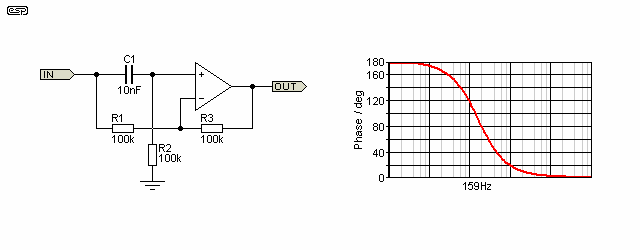Figure 9 - All-Pass Filter

The circuit shown will have a 90 degree phase shift at 159Hz.  At DC, phase shift is 180°, and at high frequencies it is 360° (note that 360° phase shift is almost the same as 0° - there is a subtle difference for transient signals, so the two can be considered identical only for steady state signal conditions).  The shift of phase about the centre frequency is completely inaudible, but if a pot is substituted for R2, the phase can be swept back and forth.  This is audible, and by cascading a number of these circuits 'phaser' or vibrato (frequency modulation) effects pedals can be made.  One of the latter is described in my projects pages.

The input signal is effectively applied to both opamp inputs, but there is always a small phase difference except at DC or infinite frequency.  The value of C1 and R2 determine the frequency at which there is a 90° (or 270°) shift, and the frequency is determined with the formula ...

fo = 1 / ( 2 × π × R2 × C1 ) ...    where fo is the 90° phase shift frequency

A quick analysis will show how this works.  Assume a DC input of 1V; at DC the cap has no effect, so the circuit operates just like an inverting buffer.  The output is therefore -1V, so there is a 180° phase shift.  At high frequencies, the reactance of C1 is negligible, and the full input is supplied to the opamp's +in terminal.  Remembering Rule #1, the opamp output will be such that both inputs will have the same voltage, and in order to do this, the output must be equal to the input at high frequencies.

At intermediate frequencies, the combination of C1 and R2, along with R1 and R3 will ensure that the output amplitude remains constant, but the phase will change.  The relative positions of C1 and R2 may be reversed, which will modify the characteristics of the circuit.

5.4 - Phase Shift Oscillator

There are many things in life I do not understand, but one of the simpler ones is the phase shift oscillator implemented using an opamp.  Don't get me wrong - the circuit I understand perfectly.  The bit I don't understand is how come (up until comparatively recently) I had never seen this circuit published - anywhere ???

In its heyday, the phase shift oscillator circuit was used almost anywhere a simple sine wave oscillator was needed, and I have seen it made with valves, transistors and even FETs.  Note that when used with a single transistor, valve or FET, the positions of the resistors and capacitors are reversed (i.e. caps are in series, with resistors to ground).  What I had not seen until I designed one was a phase shift oscillator using an opamp and using the configuration shown below.  As it transpires, although I had not seen this done, my trusty (de-facto) editor in the UK had.  This article was first published in 2000, and since then the circuit shown has been published in many web pages, but the fact remains that way back in 2000, there was no sign of it on the internet.

I have since seen it in a number of publications, including John Linsley-Hood's 'The Art of Linear Electronics'.  JLH also supplies the equation for frequency calculation ...

fo = √6 / ( 2 × π × C × R ))     Where R is resistance (R1=R2=R3) and C is capacitance (C1=C2=C3)

Loop gain must be 29.25 dB according to Linsley-Hood, and lacking further information I must assume that the formula only applies if all resistors and capacitors are equal, and gain would be the minimum required for the circuit to oscillate.  Any opamp will have sufficient gain for frequencies up to at least a few kHz.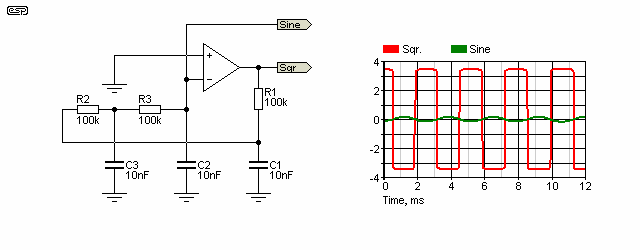Figure 10 - Phase Shift Oscillator

The frequency stability of this circuit is quite good, but as with all phase shift oscillators the amplitude varies when the frequency is changed.  Any resistor can be varied to change the frequency, and the use of a pot allows continuous variation over a 5:1 range (or more if you experiment with the component values).

This is a perfect example of an opamp being unable to obey Rule #1, and its operation is governed completely by Rule #2.  The circuit is deliberately unstable, and the opamp is always trying to play catch-up, but without success.  If it were otherwise, the circuit would stop oscillating.

The frequency is a cow to determine if different values are used for R or C, and although I believe there is a formula, it is apparently a very tedious process (I've not seen it myself).  The circuit shown above will run at about 360Hz, with a sinewave output of around 125mV (with ±5V supplies) - although JLH's formula indicates that it should oscillate at 390Hz.  If you really want to know, you will have to build one.  Changing the value of any resistor or capacitor will change distortion, frequency and amplitude.  The square wave at the output is at close to the full ± supply voltage (limited by the output circuit of the opamp).  With ±5V supplies as shown above, squarewave amplitude is about ±3.5V using a TL071 opamp.

The sinewave shown on the oscilloscope trace is obtained from the 'Sine' terminal, and the square wave is obtained from the opamp's output ('Sqr').  The string of resistors and caps acts as a phase shift network, and oscillation takes place at that frequency where there is an exact 180 degree shift, converting negative feedback into positive feedback.  The circuit is stable at DC, since it has negative feedback through the string of resistors.

Let's have a look at how it works.  Remember Rule #2?  Now have a look at the signal at the inverting input.  As you can see, the output takes the polarity of the most positive input, so when the -in terminal is positive, the output is negative.  Over a period of time based on the resistance and capacitance, the voltage on the -in terminal will fall towards zero volts, and will eventually become negative - the output promptly swings positive, and the cycle repeats.  Like all filter circuits, the resistor/ capacitor (R/C) network introduces a time delay, and it is this (plus the simple low-pass filter formed) that produces a sinewave with around 2% distortion.  By no means wonderful, but quite adequate for a number of simple applications.

The sinewave output is at relatively high impedance, and should be buffered with an opamp before use.  Any loading will alter both amplitude and frequency.

5.5 - Schmitt Trigger Oscillator

Also known as a free-running multivibrator, the Schmitt trigger oscillator is one that is much more conventional in terms of opamp designs.  Like the phase shift oscillator (indeed, like all oscillators) it is an inherently unstable circuit.  Also like the preceding example, this circuit cannot obey Rule #1 (since that would make it stable), so follows Rule #2 instead.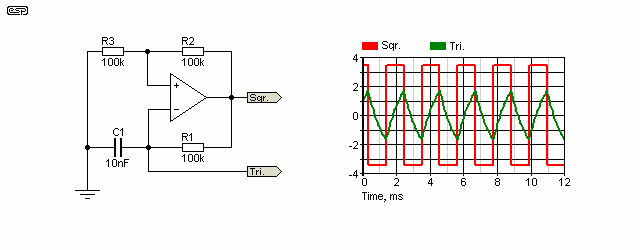Figure 11 - Schmitt Trigger Oscillator

This circuit is very common where an oscillator is needed, but as shown produces a triangular waveform that is quite high in harmonic content.  The output from the opamp is a squarewave.  Note the use of positive feedback, via R2 and R3.  This particular connection creates a Schmitt trigger, a useful but fairly inscrutable circuit for the beginner.  Although this is a simple circuit, understanding how it works is not.

Assume a supply voltage of ±5V, and we'll use the losses in the opamp output stage as shown in Figure 11.  We shall start at the point where the opamp's output is at +3.5V.  The +in terminal will be at 1.75V, since there is a voltage divider from the output to earth.  C1 will therefore charge to a positive voltage, until such time as the voltage is very slightly greater than 1.75V.  Since Rule #2 must be obeyed, the -ve input is now the more positive, so the output will swing negative.  The cap now must discharge its positive voltage and start charging to a negative voltage.  Once the negative voltage is less than (more negative than) -1.75V, the output will swing positive and the cycle repeats.  The squarewave output is ±3.5V, and the triangle wave is ±1.75V.

At least it is possible to determine the frequency of this oscillator, and it is approximately equal to ....

X = R3 / ( R2 + R3 )
fo = 1 / (2 × R1 × C1 × ln ((1 + X) / (1 - X))

For the example above (after noting that 'ln' is the natural log (base 'e'), and not base 10), frequency is ...

X = 100k / 200k = 0.5
fo = 1 / (2 × 100k × 10nF × ln (1.5 / 0.5))
fo = 1 / ( 0.002 × ln (3) )
fo = 455 Hz

The triangle wave output is at relatively high impedance, and must be buffered with an opamp before using it for anything.  Any loading will alter frequency, but not amplitude (this is fixed by the voltage divider of R2 and R3).  If the loading is too great, the oscillator will stop.  Otherwise, this is a reliable, low cost oscillator that's simple to build and is suitable anywhere that a squarewave (or buffered triangle wave) is needed.  It will run happily from a single supply, but you will need two R3 resistors, with each double the value you'd normally use.  One is wired to the +ve supply, and the other to ground, with the centre tap connected to the opamp's non-inverting (+ve) input and R2.  The resistors form a voltage divider with a nominal centre voltage of 1/2 the supply voltage.

The squarewave output rise and fall times are as fast as the opamp will allow.  If the opamp has a low slew rate (a µA741 for example) and you have a 6V peak-peak swing, the rise and fall times of the squarewave will be about 8µs.  An oscillator built with such a slow opamp will be usable up to around 4kHz.  A TL071 or similar will be quite happy up to 30kHz or more.  However, it's not a precision circuit, and it's not suitable if you need a very stable frequency.

Appendix To Part 1 (Stray Capacitance)

One thing that isn't covered above is the potential sensitivity of the inverting input to stray capacitance.  Even a small amount can create problems at high frequencies.  As a matter of course, all PCB traces that connect to the inverting input should be kept as short as possible.  If it's used as as a summing point (in a mixer for example), then the summing resistors must be close to the opamp.  This problem becomes worse with high speed opamps, and if not addressed can cause oscillation in extreme cases.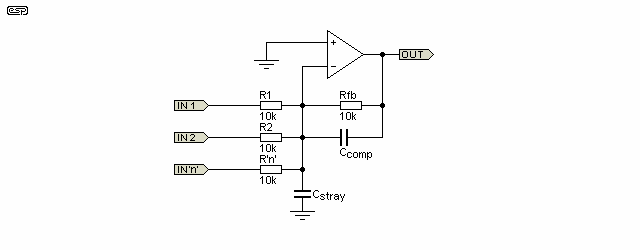Figure A1 - Input Capacitance Compensation

One way to deal with any issues caused by the capacitance is to use a capacitor in parallel with the feedback resistor (Ccomp), as shown above.  The value is determined by the capacitance at the input, the circuit gain, resistor values and is usually an unknown quantity.  All PCB traces have some capacitance, and its effect is increased if the PCB has a ground plane.  Mostly, it will be easier to arrive at a suitable feedback capacitance by experiment, because it will usually be somewhere between difficult and impossible to measure it directly.

For example, if the circuit as shown has a stray capacitance of 100pF (which would actually be quite difficult to achieve).  The compensation capacitor should be about 15pF to prevent high frequency boost and potential instability.  If one of the inputs is removed (or left floating), Ccomp needs to be greater in value - around 18pF.  Any stray capacitance from the inverting input will cause problems regardless of the circuit topology.  The above shows an inverting stage, but it's just as important for a non-inverting stage with (for example) switched resistors for gain control.

Fortunately, this is rarely an issue that anyone will come across, because sensible layout practice will ensure that the stray capacitance is low, and it's very uncommon to see any high frequency ringing with a squarewave input that's a sure indication of potential instability.  With the rather exaggerated value for Cstray shown, instability is almost a certainty, but fortunately it's rare to see more than a few pF of stray capacitance.  That usually places any problems outside the upper frequency response of the opamp.

For example, even using an 'ideal' opamp, 22pF of Cstray will cause the output to rise by 3dB at just under 2.9MHz - very few opamps have usable gain at such a high frequency, so it's not a concern.  However, if you were to examine the Project 88 circuitry, you'll see that if the first gain stage is set for 0dB gain, the feedback resistor is replaced by a link.  Stray capacitance then has no effect on the response.  There's also a warning to ensure that at least one gain switch is on, to minimise the effects of stray capacitance.

While you won't come across this particular issue often, it's something that you do need to know about.  A seemingly 'insignificant' error with PCB traces can cause issues.  If you are unaware of the potential for unwanted high frequency problems (which may include oscillation with very fast opamps), then you don't know what to look for.

Appendix 2 - µA741 Equivalent Circuit

To give you an idea of the complexity of an opamp, the following drawing shows a (roughly) equivalent circuit for the venerable Fairchild µA741.  This was the first internally compensated design, and was released in 1968.  It rapidly became a best-seller, and was easier to use than anything that had come before it.  The µA741 is quite possibly the most widely used opamp of all time, and despite its age, it's still available to this day.  The circuit shown has been simplified, and there are several versions on the Net, with most being very similar to that shown.

When first released, the most common package was a metal can (TO-99 package), as well as a ceramic 14-pin DIL package for military specifications.  I first started using them (metal can types!) in around 1972 or thereabouts, and at the time they were surprisingly expensive.  However, to get the same performance from a discrete circuit used many more parts, and would end up costing about the same as the IC anyway (especially when assembly time was factored in).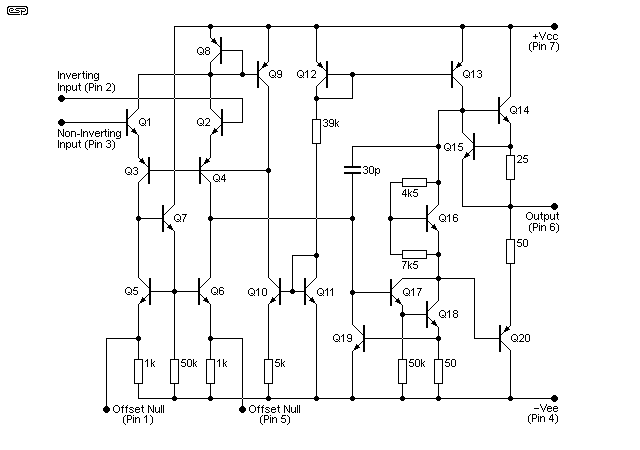Figure A2 - µA741 Equivalent Circuit

Extensive use is made of current mirrors, and the number of resistors is kept to the bare minimum.  This is because resistors are an inefficient way to use silicon, and their values can be difficult to control with any accuracy.  Because the circuit is 'equivalent', it differs from the one seen in some datasheets.  Some things that work in an IC don't work with discrete parts and vice versa.  The input stage is the expected differential pair, but uses emitter followers for the input transistors to reduce bias current and increase effective input impedance.  The remainder of the circuit provides voltage gain, and finally the short circuit protected output stage.

The thing that set the µA741 apart from earlier opamps was the compensation capacitor.  All of the previous opamps used external compensation, and making a unity-gain stable opamp with no external compensation made it instantly attractive for countless applications.  The basic architecture is also used in many other designs, most notably the 1458 - essentially a dual µA741.

During the 1970s, it was hard to find a circuit that didn't use at least one µA741, often with additional discrete parts to increase output current or reduce input noise.  Even phono preamps (with a discrete front end for lower noise) used the µA741 - not exactly ideal, but generally better than the fully discrete designs that came before it.  There are quite a few web pages available that cover the µA741 in great detail, so if you are interested in finding out more, do a web search.

One website even offers a kit to build your own fully discrete version of the µA741 - since I don't have any affiliation with the seller I'm not going to provide the URL here, but it's easily located.  Examining the circuit (especially with a simulation) is instructive, and the circuit shown above simulates very well.  Distortion is higher than the simulator's µA741 model, but otherwise performance is very similar.  It's close to impossible to build an IC circuit from discrete parts and get identical performance, because components in the IC are optimised (and very well matched where required).  This can't be done so easily with the discrete parts, so performance will suffer.  Nonetheless, it's instructive and helps your understanding of how IC opamps work.

References

I have used various references while compiling this article, with most coming from my own accumulated knowledge.  Some of this accumulated knowledge is directly due to the following publications:

IC Op-Amp Cookbook - Walter G Jung (1974), published by Howard W Sams & Co., Inc. ISBN 0-672-20969-1
Data sheets from National Semiconductor, Texas Instruments, Burr-Brown, Analog Devices, Philips and many others.
Philbrick Archive - much info on very early valve opamps, as well as later transistorised versions. Great reading !

AN166 - Basic Feedback Theory, Philips Semiconductors Application Note, Dec 1988 (See Note 1)
Opamps For Everyone - by Ron Mancini, Editor in Chief, Texas Instruments, Sep 2001

Note 1: There are errors in this document, and I have added PDF notes that explain what is wrong in each location.Articles IndexMain Index

 Copyright Notice. This article, including but not limited to all text and diagrams, is the intellectual property of Rod Elliott, and is Copyright © 2000.  Reproduction or re-publication by any means whatsoever, whether electronic, mechanical or electro- mechanical, is strictly prohibited under International Copyright laws.  The author (Rod Elliott) grants the reader the right to use this information for personal use only, and further allows that one (1) copy may be made for reference.  Commercial use is prohibited without express written authorisation from Rod Elliott.